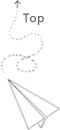# Algorithm(7) hash table and two pointers based on array (1)

### two sum (1)

Given an array of integers, find two numbers such that they add up to a specific target number.

The function twoSum should return indices of the two numbers such that they add up to the target, where index1 must be less than index2. Please note that your returned answers (both index1 and index2) are not zero-based.

You may assume that each input would have exactly one solution.

Input: numbers={2, 7, 11, 15}, target=9
Output: index1=1, index2=2

#### 解法一

• 定义Node接口体 对生成vectorvnode对其进行初始化赋值
• 对vnode 根据每个元素的value值 进行vector排序
• 采用左右两个指针 向中间靠 发现符合 node1.v+node2.v=target 的两个元素
• 和大于target则右指针左移 和小于target则左指针右移
• 取出其index值，index1=min(node1.index,node2.index) index2=max(node1.index,node2.index)

• create map < int,int > the lenth of vector is len 其中key值为array中的数字，value值为该数字在序列中的下标
• 遍历 vector 每次 keyb= nums[i] keya = target - keyb
• 如果keya不在map中，map[keya]=i
• 否则，返回 index1=map[keya] index2=map[keyb]

### 3Sum(15)

#### 解法一

• 一个是在遍历的时候 元素重复了就直接跳过
• 还有一个是 发现一组解之后 指针left right就要向两边移动 把相同的元素都跳过 就是函数中的那两个while循环 但还是要保证 left < right的
其他的细节问题，vector嵌套以及声明的时候在vc6.0中注意空格 ，比如 vector<vector > , vector temp 否则会编译错误，虽然老生常谈的了，还是被坑了。每次temp vector存数据的时候，先clear一下，貌似之前的记录还在。

### 3Sum closest

Given an array S of n integers, find three integers in S such that the sum is closest to a given number, target. Return the sum of the three integers. You may assume that each input would have exactly one solution.

• 设置left right两个指针 temp=a[left]+a[right] closet=temp
• while （left<right） 更新 temp
• if temp == target 返回 temp a[left] a[right] 是所需寻找的值
• if temp < target left ++ 如果 abs(temp-target)<abs(closet-target) closet=temp
• if temp > target right– 如果 abs(temp-target)<abs(closet-target) closet=temp
最后返回closet
注意closet的持续更新，每次都要用temp-target和closet-target来进行比较，之后更新closet,有需要的化，还需要及时记录当时的left和right的位置，因为不可能靠移动一边的指针找到距离target最近的sum，总之这里要好好体会体会并且记会，属于基本套路了。

### 3Sum smaller

Given an array of n integers nums and a target, find the number of index triplets i, j, k with 0 <= i < j < k < n that satisfy the condition nums[i] + nums[j] + nums[k] < target.

For example, given nums = [-2, 0, 1, 3], and target = 2.

Return 2. Because there are two triplets which sums are less than 2:

[-2, 0, 1]
[-2, 0, 3]

• while (i<j)
• sum3=nums[i]+nums[j]+num[now]
• if (sum3<target) ，由于序列已经是有序的，这个时候 nums[now]+nums[i]+nuns[j-1] 、nums[now]+nums[i]+nuns[j-2]… nums[now]+nums[i]+nums[i+1]这些组合都是符合要求的，所以符合要求的组合数目要加上 j-i 。之后让i后移一位。
• if (sum3>=target) 这个时候就是往小移动，即 j– 还要处理下重复的情况 如果 num[j]=nums[j-1]的时候 也前移一下 放在一个while循环中 始终保持 i<j

### 4Sum

Given an array S of n integers, are there elements a, b, c, and d in S such that a + b + c + d = target? Find all unique quadruplets in the array which gives the sum of target.

Note:
Elements in a quadruplet (a,b,c,d) must be in non-descending order. (ie, a ≤ b ≤ c ≤ d)
The solution set must not contain duplicate quadruplets.
For example, given array S = {1 0 -1 0 -2 2}, and target = 0.

### Longest Substring Without Repeating Characters

Given a string, find the length of the longest substring without repeating characters. For example, the longest substring without repeating letters for “abcabcbb” is “abc”, which the length is 3. For “bbbbb” the longest substring is “b”, with the length of 1.

• 一个while循环中 两个指针l,r初始位置都是0
• 当前字符没有出现过 ，r指针右移，更新position。
• 当前字符已经出现过 ，更新长度 ，记录position的位置，l指针移动到上次出现位置的下一个。注意限制条件，要上次的位置大于l的位置才能更新，比如 “ eccebaad ” 当遍历到第二个e的时候，position中记录的last位置为1，这个时候不要更新。
• 最后r指针都向后移动一位。

### Longest Substring with At Most Two Distinct Characters

Total Accepted: 1167 Total Submissions: 3961
Given a string, find the length of the longest substring T that contains at most 2 distinct characters.
For example,Given s = “eceba”,
T is “ece” which its length is 3.

## 推荐文章